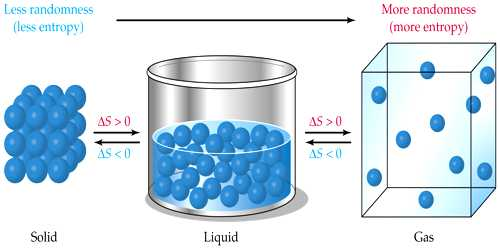Physics

# EntropyEntropy is a measure of the disorder or randomness of a system. A campfire is an example of entropy.

Scientist Clausius realized while applying second law of thermodynamics that as the temperature remains constant in an isothermal process, similarly something remains constant in an adiabatic process. In adiabatic, process when the body does not exchange heat with the surroundings then the thermal property which remains constant Clausius gave its name entropy. So, the following definition of entropy may be given:

The thermal property that remains constant in the adiabatic process is called entropy. Alternately, entropy is the physical property of the body that remains constant in an adiabatic process.

Entropy is a physical property of matter. In thermodynamics, it has tremendous importance. It is a function of the thermodynamic quantities that indicate the direction of heat flow and helps in ascertaining the thermal condition. It is a physical property of the body.

It is called thermal inertia. Entropy is a measurable quantity. How much change of entropy of a system takes place can be determined.

Like temperature, volume and pressure entropy is also a physical quantity. Its value depends on the present state of the body. But it does not depend on the path through which the body has reached to that state, i.e., at a particular state the entropy of the body does not depend on its previous history. The entropy of body changes due to absorption or rejection of heat.

Entropy is measured by the rate of change of heat absorbed or rejected with respect to the temperature of the system.

Suppose a system absorbs or rejects dQ amount of heat at absolute temperature T. So change of entropy,

dS = dQ/T

Unit: Unit of T is Kelvin and the unit of dQ is joule/kelvin JK-1